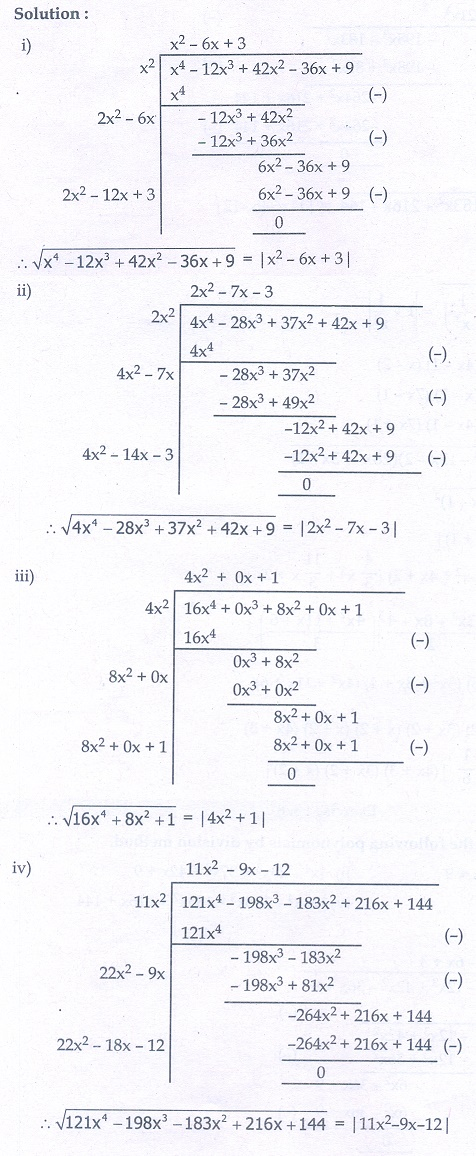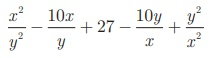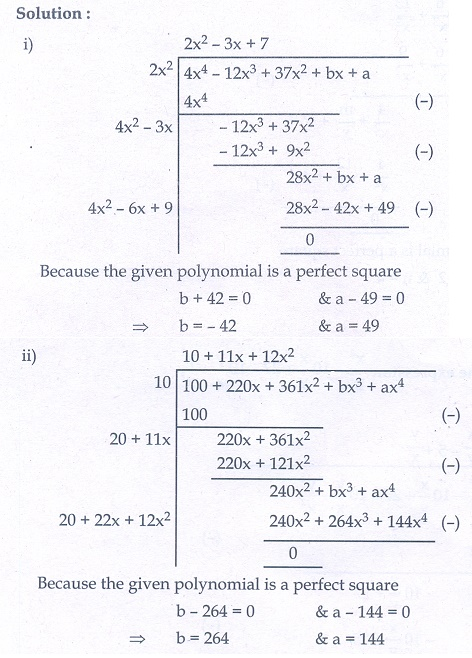Home | | Maths 10th Std | Exercise 3.8: Finding the Square Root of a Polynomial by Division Method

# Exercise 3.8: Finding the Square Root of a Polynomial by Division Method

Maths Book back answers and solution for Exercise questions - Mathematics : Algebra: Finding the Square Root of a Polynomial by Division Method: Exercise Problem Questions with Answer

### Exercise 3.8

1. Find the square root of the following polynomials by division method

(i) 4  123 + 422  36+ 9

(ii) 37x 2 − 28x 3 + 4x 4 + 42x + 9

(iii) 16x 4 + 8x 2 + 1.

(iv) 121x 4 − 198x 3 −183x 2 + 216x + 1442. Find the square root of the expression3. Find the values of a and b if the following polynomials are perfect squares

(i) 4x4 − 12x3 + 37x2 + bx +a

(ii) ax 4 + bx 3 + 361x 2 + 220x + 1004. Find the values of m and n if the following expressions are perfect squares

(i)(ii) x 4 − 8x 3 + mx2 + nx + 161.(i) |2  6+ 3| (ii) |2x 2 - 7x  3| (iii) |4x 1| (iv) | 11x 2 - 9x -12 |

2. x/y -  5 + y/x |

3.(i) 49, -42 (ii) 144, 264

4 (i) -12 , 4 (ii) 24, -32

Tags : Problem Questions with Answer, Solution | Mathematics , 10th Mathematics : UNIT 3 : Algebra
Study Material, Lecturing Notes, Assignment, Reference, Wiki description explanation, brief detail
10th Mathematics : UNIT 3 : Algebra : Exercise 3.8: Finding the Square Root of a Polynomial by Division Method | Problem Questions with Answer, Solution | Mathematics